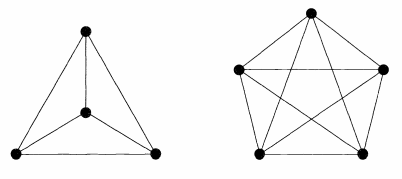# Graph Theory Basics – Part 1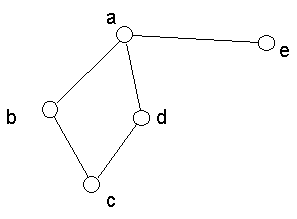1. A,B,C,D and E are vertices
2. AB, BC, CD etc are edges
3. the whole diagram is called a graph.
4. The degree of a vertex is the number of edges with that vertex as an end-point; B is degree 2, written as deg(B)5. Graphs with no loops or multiple edges, such as the graph in 1, are called simple graphs.
6. Directed graph with walks between edges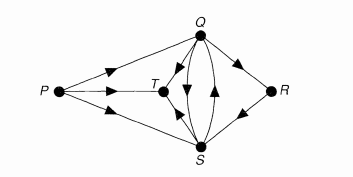7. A walk is a ‘way of getting from one vertex to another’, and consists of a sequence of edges, one following after another. For example, in above figure P —> Q—>R is a walk of length 2, and P —> S —> Q —> T —> S —> R is a walk of length 5. A walk in which no vertex appears more than once is called a path; for example, P—> T—> S —> R is a path. A walk of the form Q—> S—> T—> Q is called a cycle.
8. walks that include every edge or every vertex exactly once, ending at the initial vertex; such graphs are called Eulerian and Hamiltonian graphs.
9. Two graphs Gl and G2 are isomorphic if there is a one-one correspondence between the vertices of Gx and those of G2 such that the number of edges joining any two vertices of Gx is equal to the number of edges joining the corresponding vertices of G2.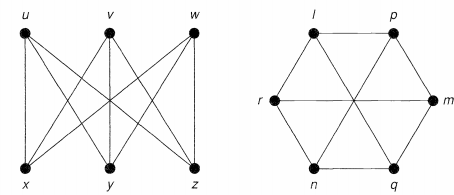10. We say that two vertices v and w of a graph G are adjacent if there is an edge vw joining them, and the vertices v and w are then incident with such an edge.
11. A graph whose edge-set is empty is a null graph.
12. Matrix representation of graphs for computer storage. If G is a graph with vertices labelled {1, 2, …,n} , its adjacency matrix A is the n x n matrix whose ij-th entry is the number of edges joining vertex i and vertex j . If, in addition, the edges are labelled {1, 2,… , m}, its incidence matrix M is the n x m matrix whose ij-th entry is 1 if vertex i is incident to edge;, and 0 otherwise.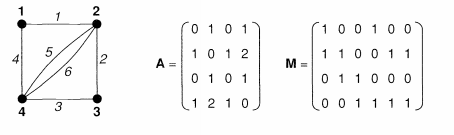13. A simple graph in which each pair of distinct vertices are adjacent is a complete graph.